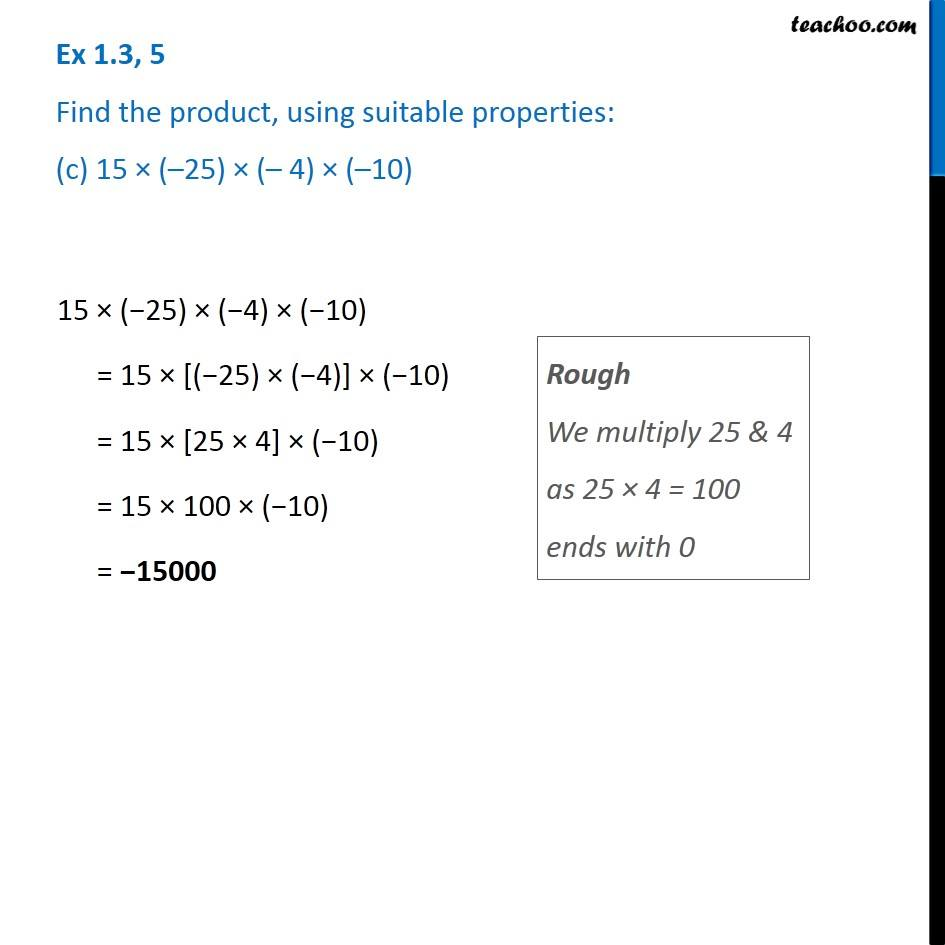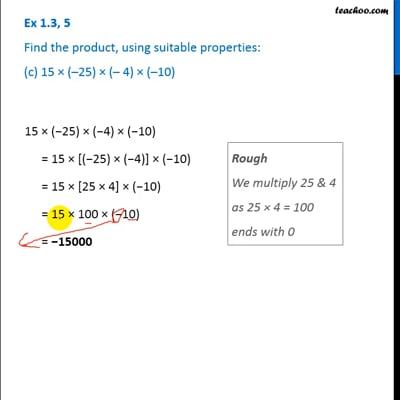Ex 1.3

Chapter 1 Class 7 Integers
Serial order wiseThis video is only available for Teachoo black users

### Transcript

Ex 1.3, 5 Find the product, using suitable properties: (c) 15 × (–25) × (– 4) × (–10) Rough We multiply 25 & 4 as 25 × 4 = 100 ends with 0 15 × (−25) × (−4) × (−10) = 15 × [(−25) × (−4)] × (−10) = 15 × [25 × 4] × (−10) = 15 × 100 × (−10) = −15000